## Sequence and Series

Subject: Optional Mathematics

#### Overview

The collection of numbers in which numbers are arranged in a certain rule is called a sequence, when the numbers of the sequence are connected by signs '+' or '-' then it is called series.

### Sequence

A Sequence is a list of things (usually numbers) that are in order. let us consider the following of numbers.

(i) 1, 4, 7, 10,.....

(ii) 20, 18, 16, 14,....

(iii) 1, 3, 9, 27, 81,....

(iv) 1, 2, 3, 4, ...

We observe that each term after the first term

(i) is formed by adding 3 to the preceding term;

(ii) is formed by subtracting 2 from the preceding term;

(iii) is formed by multiplying the preceding term by 3; each term in

(iv) is formed by squaring the natural numbers 1, 2, 3, 4,.....

In all the above case, we see that set of number follow a certain rule and we can easily say what number will come next to given number. thus, the numbers come in succession in accordance with a certain rule or low. A succession of numbers formed and arranged in a definite order according to a certain definite rule is called a sequence. the successive number in a sequence are called its terms.

### Series

A series is formed by adding or subtracting the successive term of a sequence. A series is finite or infinite according to as the number of terms added in the corresponding sequence is finite or infinite.

eg. 1 + 4 + 7 + 10 + .......... + 25 is a finite series and 2 + 4 + 6 + 8 + ........... is finite series.

The successive numbers forming the series are called the terms of the series and the successive terms are denoted by t1, t2, t3,....., t,which denotes the 1st, 2nd, 3rd, ...... nth term respectively. The nth term, tn, of a series, is called its general term. Thus, in a series, 1+4+7+10+.......+25, the first term is 1, the second term is 4, the third term is 7, and so on.

### Progression

A sequence of number is said to be a progression if the difference or ratio between its two successive terms is constant throughout the whole sequence. An example of progression is as follows.

(i) 1, 3, 5, 7,..... (ii) 1, 3, 9, 27,.....

In (i), the difference between two successive terms is equal to 2.

In (ii), the ratio of two successive terms is equal to 3.

### Types of Progression

Progression is divided into following two types.

(i) Arithmetic progression

(ii) Geometric progression

#### Arithmetic progression or Sequence

A sequence is called an arithmetic progression if the difference between its two successive terms is constant throughout the whole sequence. An arithmetic progression can be denoted by A.P. The constant number obtained by subtracting succeeding term from its preceding term is called the common difference.

For example:-

(i) 1, 3, 5, 7, 9,....

(ii) 15, 12, 9, 6,......

From (i), we find that

second term - first term = 3 -1 = 2,

third term - second term = 5 - 3 = 2,

fourth term - third term = 7 - 5 = 2 and so on.

From (ii), we find that

second term - first term = 12-15 = -3,

third term - second term = 9 - 12 = -3,

fourth term - third term = 6 - 9 = -3 and so on.

Hence, the common difference 'd' is calculated by

d =  succeeding term - proceeding term = tn - tn-1

Here, we find that the difference between two successive terms, in both sequences, are same or constant. So, such sequence is called arithmetic progressions. The C.D. of the two progressions are 2 and -3 respectively. Thus, arithmetic progressions is a series in which the successive terms increase or decrease by the common difference.

##### General term or nth term of an A.P.

To find the nth term of an A.P.

Let, t1 be the first term, n be the number of terms and 'd' the common difference of an A.P. respectively. Then,

t= a = a + (1-1)d

t= a + d = a + (2-1)d

t= a + 2d = a + (3-1)d

t= a + 3d = a + (4-1)d

In general, tn = a + (n-1)d

Formula: If tdenotes the nth term, of the arithmetic progression whose first term, common term and number of terms are a, d and n respectively.

With this term, arithmetic sequence and series can be written as:

Arithmetic sequence: a, a+d, a+2d, a+3d, ............

Arithmetic series: a+ (a+d) + (a+2d) + (a+3d), ..........

#### Arithmetic Mean

The terms between the arithmetic progression are known as arithmetic mean. Such as the three numbers 2, 4, 6 are in arithmetic progression with the common difference d = 2, then 4 is the arithmetic mean between 2 and 6.

For example:

Let a, b,c are in arithmetic progression

b-a = c-b

or, b+b = a+c

or, 2b = a+c

or, b = $\frac{a+c}{2}$

Hence the arithmetic mean between a and c is ($\frac{a+c}{2}$)

n Arithmetic Means between two numbers a and b

Let m1, m2, m3, .........mn be the arithmetic means between the given term a and b. Then, a, m1, m2, m3, .........mn, b are in A.P.

Here, numbers of arithmetic means = n

So, numbers of terms of A.P. = n+2

It means,

b = (n+2)th term of AP

or, b = a + (n+2-1)d, where d is common difference

or, b =a + (n+1)d

or, (n+1)d = b-a

∴ d= $\frac{b-a}{n+1}$

Now, m1 = a+d = a +  $\frac{b-a}{n+1}$

m2 = a + 2d = a +  $\frac{2(b-a)}{n+1}$

m3 =  a +  $\frac{3(b-a)}{n+1}$

.............................................

mn =  a +  $\frac{n(b-a)}{n+1}$

#### Sum of n terms of series in A.P.

Let us consider an arithmetic series

a + (a+d) + (a+2d) + (a+3d) + ...... + (l-2d) +(l-d) + l

Here, the first term = a,

first term = a,

common difference = d,

number of terms=  n,

last term (tn) = l

the term before last term = l-d

if the sum of n terms is denoted by Sn, then

Sn = a + (a+d) + (a+2d) + (a+3d) + ...... + (l-2d) +(l-d) + l .... (i)

Writing term in the reverse order,

Sn = l + (l-d) + (l-2d)  + ...... + (a+3d) + (a+2d) + (a+d) + a .... (ii)

Adding the corresponding terms of (i) and (ii)

$\frac{S_n \;= \;a \;+\; (a+d)\; +\; (a+2d)\; +\; (a+3d)\; + \;......\; + \;(l-2d) \;+\; (l-d)\; + \;l\\S_n\;=\;l\; +\;(l-d)\;+\;(l-2d)\;+\;......\;+\;(a+3d)\;+\;(a+2d)\;+\;(a+d)\;+a\:}{2S_n\;= \;(a+l) \;+ \;(a+l)\; + \;(a+l)\; +\; ............ \;+\; (a+l)\; + \;(a+l)\; +\; (a+l)}$

=   n times (a+l)

=   n (a+l)

= $\frac{n}{2}$(a+l)

But, the last term l = a + (n-1)d

So, Sn = $\frac{n}{2}$(a+l) = $\frac{n}{2}$[a+a+(n-1)d] = $\frac{n}{2}$[2a+(n-1)d]

∴ Sn =  $\frac{n}{2}$[2a+(n-1)d]

Thus, if d is unknown, Sn = $\frac{n}{2}$(a+l)

And, if l is unknown, Sn = $\frac{n}{2}$[2a+(n-1)d]

1. Sum of first n natural numbers

the numbers 1, 2, 3, 4, ......, n are called the first n natural numbers.

Here, first term (a) = 1

Common difference (d) = 2-1 = 1

Number of terms (n) = n

If Sn denotes the sum of these first n natural numbers, then

Sn = $\frac{n}{2}$[2a+(n-1)d] = $\frac{n}{2}$[2.1+(n-1).1] =  $\frac{n}{2}$[2+n-1] = $\frac{n}{2}$(n+1)

2. Sum of first n odd numbers

1, 3, 5, 7, ......., (2n-1) are the first n odd numbers.

Here, first term (a) = 1

Common difference (d) = 3-1 = 2

Number of terms (n) = n

If Sn denotes the sum of these first n odd numbers, then

Sn = $\frac{n}{2}$[2a+(n-1)d] = $\frac{n}{2}$[2.1+(n-1).2] =  $\frac{n}{2}$(2+2n-2) =  $\frac{n}{2}$ × 2n = n2

3. Sum of first n even numbers

2, 4, 6, 8, ......., 2n are the first n even numbers.

Here, first term (a) = 2

Common difference (d) = 4-2 = 2

Number of terms (n) = n

If Sn denotes the sum of these first n even numbers, then

Sn = $\frac{n}{2}$[2a+(n-1)d] = $\frac{n}{2}$[2.2+(n-1).2] =  $\frac{n}{2}$(4+2n-2) =  $\frac{n}{2}$(2n-2) = n(n+1)

##### Geometric Progression or Sequence

In mathematics, a geometric progression, also known as a geometric sequence, is a sequence of numbers where each term after the first is found by multiplying the previous one by a fixed, non-zero number called the common ratio. For example, the sequence 2, 6, 18, 54, ... is a geometric progression with common ratio 3. Similarly,10, 5, 2.5, 1.25, ... is a geometric sequence with common ratio 1/2.

Examples of a geometric sequence are powers rk of a fixed number r, such as 2and 3k. The general form of a geometric sequence is

a, ar, ar2, ar3, ............arn

where r ≠ 0 is the common ratio and a is a scale factor equal to the sequence's start value.

General Term or nth term of G.P.

We use the following notations for terms and expression involved in a geometrical progression:

The first term = a the nth term = toor b

The number of terms = n Common ratio = r.

The expression arn-1 gives us the nth term or the last term of the geometric progression whose first term, common ratio and a number of terms are a, r and n respectively.

∴tn = arn-1

With the help of this general term, geometric sequence and series can be written in the following ways:

Geometric Sequence: a, ar, ar2, ar$^3$, .....

Geometric series: a + ar + ar2 + ar$^3$ + ........

#### Geometric Mean

If the three numbers are in G.P., then the middle term is called the geometric mean of the other two terms. In other words, the geometric mean of two non-zero numbers is defined as the square root of their product.

Let a, G, b be three numbers in G. P., then the common ratio is the same i.e.

$\frac{G}{a}$ =$\frac{b}{G}$

or, G2 = ab

or, G =$\sqrt{a}{b}$

Hence, the geometric mean of two numbers a and b is the square root of their product i.e. $\sqrt{a}{b}$.

So, the geometric mean between two number 2 and 8 is G =$\sqrt ab$ = $\sqrt2*8$ = $\sqrt16$ = 4.When

When any number of quantities are in G. P., all the terms in between the first and last terms are called the geometric means between these two quantities.

Here, Gn = arn = a $\begin{pmatrix}b\\a\\ \end{pmatrix}$$\frac{n}{n + 1}$

####"Arithmetic mean (A. M) is always greater than Geometric mean (G. M.) between two position real unequal numbers".

Let us consider two numbers 2 and 8

Here, AM between 2 and 8 =$\frac{2 + 8}{2}$ = 5

GM between 2 and 8 = $\sqrt 2 * 8$) = 4

∴ AM > GM.

The sum of n terms of a series in G. P.

Let us consider geometric series a + ar + ar2 + ar$^3$ + .......+ arn -3+ arni2+ arn-1

Here, first = a common ratio = r number of terms = n last term (l) = arn-1

∴ Sn = $\frac{lr - a}{r - 1}$

If the number of terms is odd, we take the middle term as aand the common ratio as r. If the number of terms is even, we take $\frac{a}{r}$ and ar as the middle terms and r2 as the common ratio.

##### Things to remember

A series is formed by adding or subtracting the successive term of a sequence. A series is finite or infinite according to as the number of terms added in the corresponding sequence is finite or infinite.

eg. 1 + 4 + 7 + 10 + .......... + 25 is a finite series and 2 + 4 + 6 + 8 + ........... is finite series.

The successive numbers forming the series are called the terms of the series and the successive terms are denoted by$$t_1, t_2, t_3,....., t_n,$$ where$$t_1, t-2, t-3, ........ tn$$ denote the$$1^st, 2^nd, 3^rd ,...... .n^{nt}$$ term respectively. The n^th term, t_n, of a series, is called its general term. thus, in a series, 1+4+7+10+.......+25, the first term is 1, the second term is 4, the third term is 7, and so on.

The terms  between the first term and last term of an A.P are called arithmetic mean.,

• It includes every relationship which established among the people.
• There can be more than one community in a society. Community smaller than society.
• It is a network of social relationships which cannot see or touched.
• common interests and common objectives are not necessary for society.
##### Videos for Sequence and Series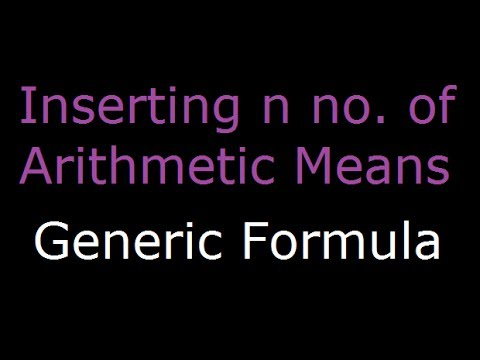##### Arithmetic Progression - n number of arithmetic means between two numbers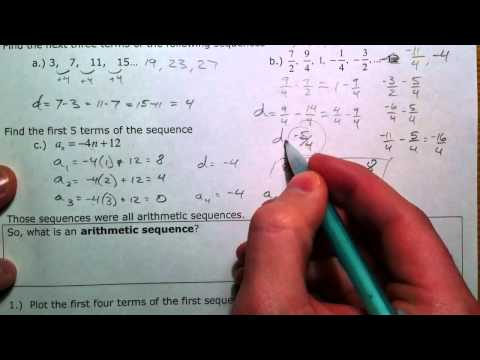##### Arithmetic Sequences and Series (1 of 3)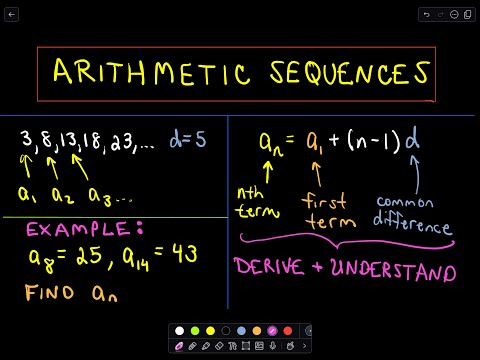##### Arithmetic Sequences: A Formula for the ' n - th ' Term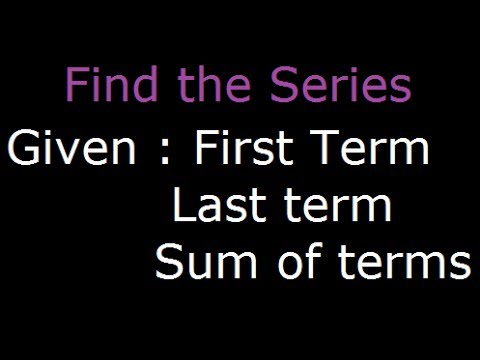##### Find Arithmetical Sequence - Given first term, last term and sum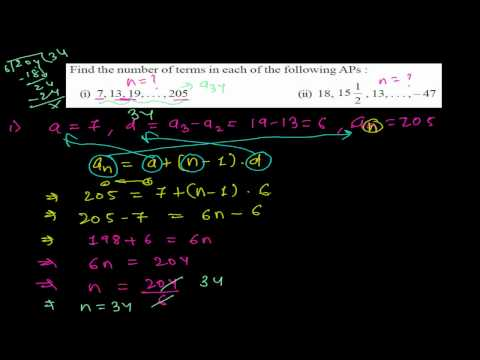##### Find Total Number of Terms of an Arithmetic Sequence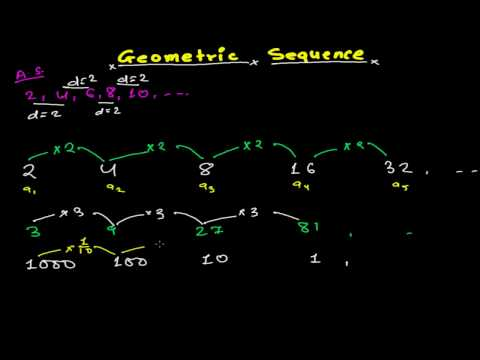##### Geometric Sequences -Geometric Series - Geometric Progression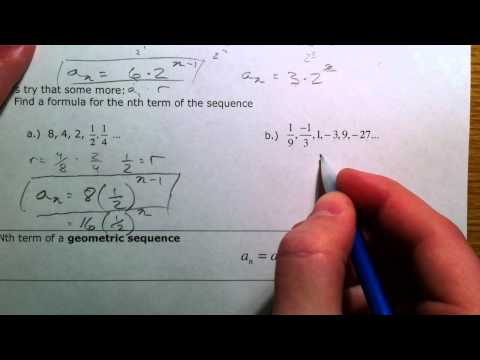##### Geometric Sequences and Series (1 of 2)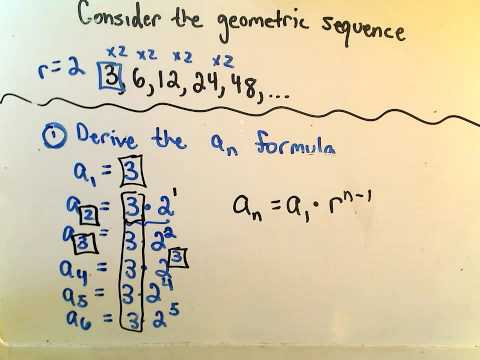##### Geometric Sequences: A Formula for the' n - th ' Term.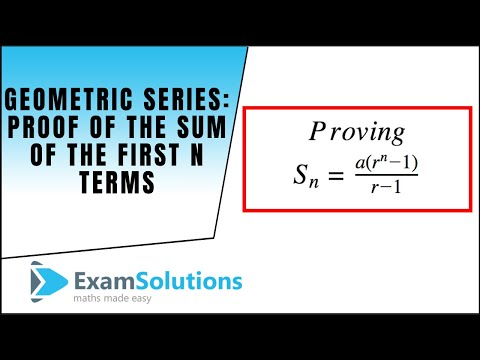##### Geometric Series - Proof of the Sum of the first n terms : ExamSolutions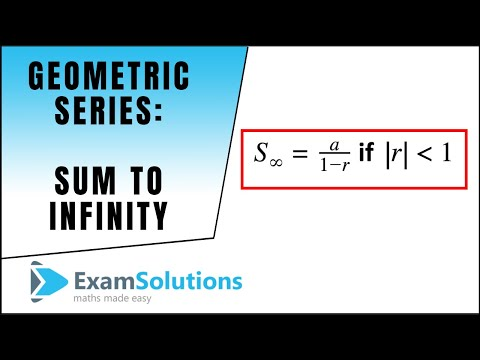##### Geometric Series - Sum to Infinity : ExamSolutions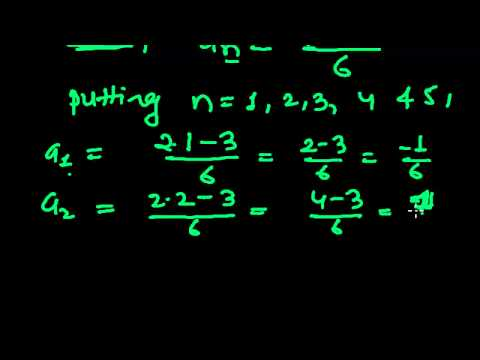##### How to Find First Five(5) Terms in Arithmetic Sequence - Sequences and Series Problems 1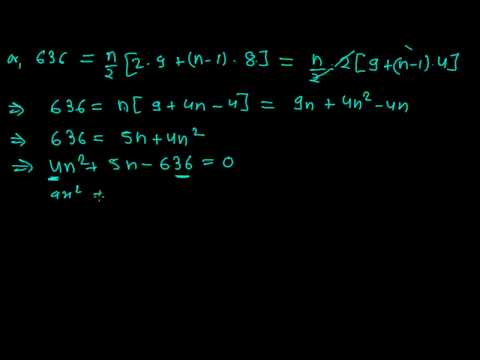##### How to find the Number of Terms of an Arithmetic Sequence from Sum of N terms Formula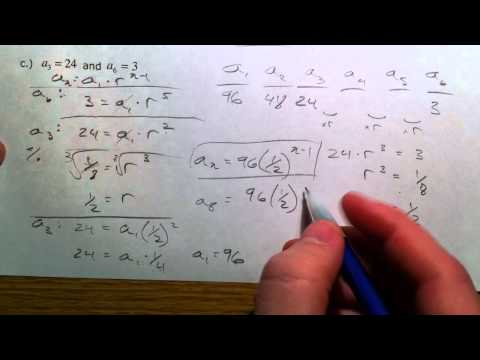$\sum_{n=2}^5 (n^2- 3)$

Putting n = 2, 3, 4, 5

= (22 - 3) + (32 - 3) + (42 - 3) + (52 - 3)

= (4 - 3) + (9 - 3) + (16 - 3) + (25 - 3)

= 1 + 6 +13 +22

= 42 Ans

un + 1 = 1 - $\frac {1}{u_n}$ and u1 = 3, u2 and u3 = ?

If n = 1,un + 1 = 1 - $\frac {1}{u_n}$

u1 + 1 =1 - $\frac {1}{u_1}$

or, u2 = 1 - $\frac 13$ = $\frac {3 - 1}{3}$ = $\frac 23$

If n = 2, un + 1 =1 - $\frac {1}{u_n}$

u2 + 1 =1 - $\frac {1}{u_2}$

or, u3 = 1 - $\cfrac{1}{\cfrac{2}{3}}$ = 1 - $\frac 32$ = $\frac {2 - 3}{2}$ = -$\frac 12$

∴ u2 = $\frac 23$ and u3 = -$\frac 12$ Ans

Sequence: A sequence is a set of numbers or quantities, which are formed according to some governed laws.

i.e. 1, 4, 16 ............................ and 2, 3, 4, 5...................

Series: The sum of the term of a sequence is called a series.

i.e. 1 + 4 + 16 + ....................... and 2 + 3 + 4 + 5 + ......................

Here,

$\sum_{n=4}^7 (3n- 2)$

where: n = 4, 5, 6, 7

$\sum_{n=4}^7 (3n- 2)$

= (3×4-2) + (3×5-2) +(3×6-2) +(3×7-2)

= (12-2) + (15-2) + (18-2) + (21-2)

= 10 + 13 + 16 + 19

= 58 Ans

$\sum_{k=3}^7 (k^2 + 1)$

= (32 + 1) +(42 + 1) +(52 + 1) +(62 + 1) +(72 + 1)

= (9 + 1) +(16 + 1) +(25 + 1) +(36 + 1) +(49 + 1)

= 10 + 17 + 26 + 37 + 50

= 140 Ans

Here,

First term (a) = 1

Second term (b) = 4

Common Difference (d) = b - a = 4 - 1 = 3

Last term (l) = 34

We know,

last term (l) = a + (n - 1)d

or, 34 = 1 + (n - 1) 3

or, 34 = 1 + 3n - 3

or, 3n - 2 = 34

or, 3n = 34 + 2

or, n = $\frac {36}{3}$

∴ n = 12

Again,

Sn = $\frac n2$ [a + l]

Sn = $\frac{12}{2}$ [1 + 34] = 6× 35 = 210

∴ Sn = 210 Ans

Here,

first term (a) = 2

second term (b) = 7

common difference (d) = b - a = 7 - 2 = 5

number of items (n) = 16

sum (S16) = ?

We know that,

Sn = $\frac n2$ [2a + (n - 1) d]

S16 = $\frac {16}{2}$ [2 × 2 + (16 - 1) 5] = 8 [4 + 15× 5] = 8 [4 + 75] = 8 × 79 = 632 Ans

Here,

first term (a) =3$\frac12$ = $\frac 72$

second terrn (b) = 1

common difference (d) = b - a = 1 - $\frac 72$ = $\frac {2 - 7}{2}$ = -$\frac 52$

no. of items (n) = ?

last term (l) =-21$\frac 12$ = -$\frac {43}{2}$

We know that,

l = a + (n - 1) d

or,-$\frac {43}{2}$ = $\frac 72$ + (n - 1) (-$\frac 52$)

or, - 43 = 7 - 5n + 5

or, 5n = 12 + 43

or, n = $\frac {55}{5}$ = 11

∴-21$\frac 12$ is the 11th term. Ans

Here,

first term (a) = 20

common difference (d) = -3

numbers of term (n) = 7

seventh term (t7) = ?

We know that,

tn= a + (n - 1) d

t7 = 20 + (7 - 1) (-3) = 20 + 6 (-3) = 20 - 18 = 2 Ans

Here,

first term (a) = 2

second term (b) = 4

common difference (d) = b - a = 4 - 2 = 2

no. of terms (n) = 20

sum (S20) = ?

We know that,

Sn = $\frac n2$ [2a + (n - 1) d]

S20 = $\frac {20}{2}$ [2× 2 + (20 - 1)× 2] = 10 [4 + 38] = 10× 42 = 420 Ans

Here,

first term (a) = 1

second term (b) = 3

common difference (d) = b - a = 3 - 1 = 2

number of items (n) = 20

twenty term (t20) = ?

We know,

tn= a + (n - 1) d

t20 = 1 + (20 - 1) 2

t20 = 1 + 19× 2 = 1 + 38 = 39 Ans

Here,

first term (a) = 25

second term (b) = $\frac {45}{2}$

common difference (d) = b - a = $\frac {45}{2}$ - 25 = $\frac {45 - 50}{2}$ = -$\frac 52$

last term (l) = - 15

number of terms (n) = ?

We know that,

l = a + (n - 1) d

or, - 15 = 25 + (n - 1) - $\frac 52$

or, - 15 - 25 = -$\frac 52$n + $\frac 52$

or, - 40 - $\frac 52$ = -$\frac 52$n

or - $\frac {80 - 5}{2}$ = -$\frac {5n}{2}$

or, -$\frac {85}{2}$× -$\frac {2}{5}$ = n

∴ n = 17

∴ The number of term = 17. Ans

Here,

first term (a) = 3

last term (l) = -9

number of terms (n) = 4 [including a and b]

common difference (d) = $\frac {b - a}{n - 1}$ = $\frac {-9 - 3}{4 - 1}$ = -$\frac {12}{3}$ = - 4

x = a + d = 3 - 4 = - 1

y = a + 2d = 3 + 2× -4 = 3 - 8 = - 5

∴ x = -1 and y = -5 Ans

Here,

first term (a) = 2

second term (b) = - 9

common difference (d) = b - a = -9 - 2 = -11

last term (l) = -130

number of terms (n) = ?

We know,

l = a + (n - 1) d

or, -130 = 2 + (n - 1) (-11)

or, -130 - 2 = - 11n + 11

or, -132 - 11 = -11n

or, -11n = -143

or, n = $\frac {-143}{-11}$

∴ n = 13

Again, Sn = $\frac n2$ (a + 1) = $\frac {13}{2}$ (2 - 130) = 6.5× -128

∴ Sn = -832 Ans

Here,

common difference (d) = -3

number of terms (n) = 7

sum (S7) = 0

first term (a) = ?

We know,

Sn = $\frac n2$ [2a + (n - 1) d]

or, 0 = $\frac 72$ [2a + (7 - 1) (-3)]

or, 0× $\frac 27$ = 2a + 6× (-3)

or, 0 = 2a - 18

or, 2a = 18

or, a = $\frac {18}{2}$ = 9

∴ first term (a) = 9 Ans

Here,

first term (a) = $\frac 14$

second term (b) = $\frac 12$

common ratio (r) = $\frac ba$ =$\cfrac{\frac{1}{2}}{\cfrac{1}{4}}$ = $\frac 12$× $\frac 41$ = 2

last term (l) = 128

number of terms (n) = ?

We know that,

l = arn-1

or, 128 = $\frac 14$ (2)n-1

or, 27× 22 = 2n-1

or, 2n-1 = 29

or, n -1 = 9

or, n = 9 + 1

∴ n = 10

∴ The number of term = 10 Ans

Here,

first term (a) = 16

last term (l) = 36

We know that,

geometric mean (G.M.) =$\sqrt {ab}$

G.M. =$\sqrt{({16}×{36})}$

= $\sqrt {({4^2}×{6^2})}$

= $\sqrt {({4×6})^2}$

= $\sqrt {({24})^2}$

∴ Geometric mean = 24 Ans

Here,

first term (a) = $\frac 13$

second term (b) = 1

common ratio (r) = $\frac ba$ = $\cfrac {1}{\cfrac 13}$ = 3number of terms (n) = 8

number of terms (n) = 8

eighth term (t8) = ?

Using formula,

tn = arn-1

t8 = ($\frac 13$) (3)8-1 = $\frac 13$× 3× 36 = 729 Ans

Here,

first term (a) = 3

second term (b) = 1

common ratio (r) = $\frac ba$ = -$\frac 13$

last term (l) = -$\frac {1}{81}$

number of terms (n) = ?

We know that,

l = arn-1

or, -$\frac {1}{81}$ = 3 (-$\frac 13$)n-1

or, - $\frac {1}{3^4× 3}$ = (-$\frac 13$)n-1

or, (-$\frac 13$)5 =(-$\frac 13$)n-1

or, n - 1 = 5

or, n = 5 + 1

∴ n = 6

∴ The number of term = 6 Ans

Here,

first term (a) = $\frac 23$

second term (b) = -$\frac 16$

common ratio (r) =$\frac ba$ = $\cfrac{\frac{-1}{6}}{\cfrac{2}{3}}$= -$\frac 16$ × $\frac 32$ = -$\frac 14$

number of items (n) = 5

5th term (t5) = ?

We know that,

tn= arn-1

or, t5 = $\frac 23$(-$\frac 14$)5-1

or, t5 = $\frac 23$×(-$\frac 14$)4

or, t5 = $\frac 23$× $\frac {1}{16}$×$\frac {1}{16}$ = $\frac {1}{384}$

∴ fifth term = $\frac {1}{384}$ Ans

Here,

first term (a) = $\frac 23$

second term (b) = x

third term (c) = $\frac 32$

We know that,

G.M. = $\sqrt {ab}$

x = $\sqrt {(\frac 23) × (\frac 32)}$

x = 1

r = $\frac ba$ =$\cfrac{1}{\cfrac{2}{3}}$ = $\frac 32$

y = ar = $\frac 32$× $\frac 32$ = $\frac 94$

∴ x = 1 and y = $\frac 94$ Ans

Here,

first term (a) = 12

second term (b) = 4 common ratio (r) = $\frac ba$ = $\frac 42$ = 2

number of terms (n) = 10

sum of the ten terms (S10) = ?

We know that,

Sn = $\frac {a(r^n - 1)}{r - 1}$ = $\frac {2(2^10 - 1)}{2 - 1}$ =$\frac {2(1024 - 1)}{1}$ = 2× 1023 = 2046

∴ The sum of tenth terms = 2046 Ans

Here,

first term (a) = 81

second term (b) = 27

common ratio (r) = $\frac ba$ = $\frac {27}{81}$ = $\frac 13$

number of terms (n) = 5

sum of five terms (S5) = ?

We know,

Sn = $\frac {a(r^n - 1)}{r - 1}$

S5 = $\frac {81[(\frac 13)^5 - 1]}{\frac 13 - 1}$

=$\frac {81[(\frac {1}{243}) - 1]}{\frac {1 - 3}{3}}$

=$\frac {81 × (\frac {1 - 243}{243})}{\frac {-2}{3}}$

= 81× -$\frac {242}{243}$× -$\frac 32$

= 121

∴ The sum of five terms = 121 Ans

Let,

first term = a

common ratio = r

From equation,

S6 = 9 S3

or,$\frac {a(r^6 - 1)}{r - 1}$ = 9 [$\frac {a(r^3 - 1)}{r - 1}$] [$\because$Sn =$\frac {a(r^n - 1)}{r - 1}$]

or, $\frac {a[(r^3)^2 - (1)^2]}{r - 1}$×$\frac {r - 1}{a(r^3 - 1)}$ = 9

or, $\frac{(r^3 + 1)(r^3 - 1)}{r^3 - 1}$ = 9

or, r3 = 9 - 1

or, r3 = 8

or, r3 = 23

∴ r = 2

∴ The common ratio = 2 Ans

Let: the three terms of an G.P. are $\frac ar$, a and ar

From Question,

$\frac ar$× a× r = 729

or, a3 = 93

∴ a = 9

∴ The second term = 9 Ans

Here,

first term (a) = 6

third term (b) = x

A.M. = 30

A.M. = $\frac {a + b}{2}$

or, 30 = $\frac {6 + x}{2}$

or, 60 - 6 = x

∴ x = 54

G.M. = $\sqrt {ab}$

G.M. = $\sqrt {6 × 54}$

G.M. = $\sqrt {2^3 × 3^3 ×3^3}$

G.M. = 18

∴ The G.M. = 18 Ans

Here,

first term (a) = $\frac 19$

last term (l) = 9

number of Geometric mean (n) = 3

total number of term (n) = 5

3 Geometric mean (m1), (m2), (m3) = ?

l = arn-1

or, 9 = $\frac 19$r5-1

or, 81 = r4

or, 34 = r4

∴ r = 3

m1 = ar = $\frac 19$× 3 = $\frac 13$

m2 = ar2 = $\frac 19$× 32 = $\frac 19$× 9 = 1

m3 = ar3 = $\frac 19$× 33 = $\frac 19$× 27 = 3

∴ m1 = $\frac 13$, m2 = 1, m3 = 3 Ans

If M and G be the arithmetic mean and geometric mean between two positive numbers a and b, then

m = $\frac {a + b}{2}$ .................... (1)

G = $\sqrt {ab}$ ................................(2)

Equation (1) - (2) we get

M - G = $\frac {a + b}{2}$ - $\sqrt {ab}$

= $\frac {a + b - 2\sqrt{ab}}{2}$

= $\frac {(\sqrt a)^2 - 2\sqrt a ⋅ \sqrt b + (\sqrt b)^2}{2}$

= $\frac 12$ ($\sqrt a$ - $\sqrt b$)2

$\sqrt a$ - $\sqrt b$ gives positive value

∴ M - G = $\frac {(\sqrt a - \sqrt b)^2}{2}$≥ 0

M - G≥ 0 or, M ≥G

∴ A.M.≥ G.M. Ans

Let:

Sn = 1, 3, 5, ..................... n

First term (a) = 1

Second term (b) = 3

Common difference (d) = b - a = 3 - 1 =2

number of items = n

We know,

Sn = $\frac n2$[2a + (n - 1) d]

or, Sn = $\frac n2$[ 2× 1 + (n - 1) 2]

or, Sn = $\frac n2$[2 + 2n - 2]

or, Sn = $\frac n2$× 2n

∴ Sn = n2Ans

Here,

first term (a) = 1

common ratio (r) = 2

sum (Sn) = 255

We know,S

Sn = $\frac{a(r^n - 1)}{r - 1}$

or, Sn =$\frac{1(2^n - 1)}{2 - 1}$

or,$\frac{2^n - 1}{1}$ = 255

or, 2n = 255 + 1

or, 2n = 256

or, 2n = 28

∴ n = 8

∴ The no. of terms = 8 Ans

Let:

first term (a) = 3

last term (b) = 243

no. of geometric means (n) = 3

common ratio (r) = ?

3 G.M are X1, X2, X3 = ?

r = ($\frac ba$)$\frac {1}{n+1}$= ($\frac {243}{3}$)$\frac {1}{3+1}$= (81)$\frac 14$= (3)$\frac {4×1}{4}$ = 3

X1 = ar = 3× 3 = 9

X2 = ar2 =3× (3)2 = 27

x3 = ar3 =3× (3)3 = 81

∴ 9, 27, 81 Ans

Let:

first term (a) = 27

last term (l) = $\frac {32}{9}$

number of terms (n) = 4

common ratio (r) = ($\frac ba$)$\frac {1}{n+1}$

= ($\cfrac{\frac{32}{9}}{27}$)$\frac {1}{4+1}$

=( $\frac {32}{9×27}$)$\frac 15$

= ($\frac 23$)$\frac {5×1}{5}$

=$\frac 23$

p = ar = 27×$\frac 23$ = 18

q = ar2=27×$\frac 23$×$\frac 23$ = 12

r = ar3 =27×$\frac 23$×$\frac 23$×$\frac 23$ = 8

s = ar4 =27×$\frac 23$×$\frac 23$×$\frac 23$×$\frac 23$ = $\frac {16}{3}$

∴ p = 18, q = 12, r = 8 and s = $\frac {16}{3}$ Ans

Here,

first term (a) = 2

second term (b) = -6

common ratio (r) = $\frac ba$ = $\frac {-6}{2}$ = -3

number of terms (n) = 6

We know that,

Sn = $\frac {a(1 - r^n)}{1 - r}$

Sn = $\frac {2(1 - (-3)^6)}{1 - (-3)}$

Sn = $\frac {2(1 -729)}{1 + 3}$

Sn = $\frac {2× (-729))}{4}$

∴Sn= -364.5Ans

Here,

first term (a) = 3

second term (b) = 6

common ratio (r) = $\frac ba$ =$\frac 63$ = 2

last term (l) = 1535

sum of the series (Sn) = ?

Using formula,

Sn = $\frac{lr - a}{r - 1}$ = $\frac {1535 × 2 - 3}{2 - 1}$ = $\frac {3070 - 3}{1}$ = 3067

∴ Sum (Sn) = 3067 Ans

Let:

first term = a

common difference = d

number of terms (n) = 4

sum of 4 terms (S4) = 26

We know that,

Sn = $\frac n2$ [2a + (n - 1) d]

or, S4= $\frac 42$ [2a + (4 - 1) d]

or, 26 = 2[2a + 3d]

or, 2a + 3d = 13 ........................(1)

Again,

number of terms (n) = 8

sum of 8 terms (S8) = 100

Sn = $\frac n2$ [2a + (n - 1) d]

or, S8= $\frac 82$ [2a + (8 - 1) d]

or, 100 = 4[2a + 7d]

or, 2a + 7d = 25 ........................(2)

Subtracting the equation (1) from equation (2)

 2a + 7d = 25 2a + 3d = 13 - - - 4d = 12

or, d = $\frac {12}{4}$

∴ d = 3

Substituting the value of d in equation (2)

2a + 7d = 25

or, 2a + 7× 3 = 25

or, 2a = 25 - 21

or, a = $\frac 42$

∴ a = 2

Sum of 28 terms (S28) = ?

Sn = $\frac n2$ [2a + (n - 1) d]
or,S28 = $\frac {28}{2}$ [2(2) + (28 - 1) d]

or,S28 = 14[4 + 27× 3]

or,S28 = 14 (4 + 81)

or,S28 = 14× 85

∴S28 = 1190 Ans

Here,

first term (a) = 27

second term (b) = 24

common difference (d) = b - a = 24 - 27 = -3

Sum of the series (Sn) = 132

We know that,

Sn = $\frac n2$ [2a + (n - 1) d]

or, 132 = $\frac n2$ [2×27 + (n - 1) (-3)]

or, 264 = n[52 - 3n + 3]

or, 264 = n(57 - 3n)

or, 264 = 57n - 3n2

or, 3n2 - 57n + 264 = 0

or, 3(n2 - 19n + 88) = 0

or, n2 - 11n - 8n + 88 = 0

or, n(n - 11) -8(n - 11) = 0

or, (n - 11) (n - 8) = 0

Either, n - 11 = 0 ∴ n = 11

Or, n - 8 = 0 ∴ n = 8

∴n = 11 or 8

∴ The double answer of n show that there are two series in A.S. having the same sum.

The series to 8 terms 27 + 24 + 21 + 18 + 15 + 12 + 9 + 6 and the series to 11 terms is27 + 24 + 21 + 18 + 15 + 12 + 9 + 6 + 3 + 0 - 3

∴ S8 = S11 =132 Ans

Here,

third term (t3) = 40

number of terms (n) = 3

Using formula,

tn= a + (n - 1)d

t3 = a + (3 - 1)d

40 = a + 2d ............................(1)

Again,

thirteen term (t13) = 0

number of terms (n) = 13

Using formula,

tn= a + (n - 1)d

t13 = a + (13 - 1)d

0 = a + 12d ...........................(2)

Subtracting equation (2) from (1)

 a + 2d = 40 a + 12d = 0 - - - - 10d 40

∴ d = -4

Substituting the value of d in equation (2)

a + 12d = 0

a + 12× (-4) = 0

a - 48 = 0

∴ a = 48

Now,

a = 48

d = -4

n = 25

t25 = ?

We know,

tn= a + (n - 1)d

∴ t25 = 48 + (25 - 1)(-4) = 48 + 24 (-4) = 48 - 96 = - 48 Ans

Let:

first term = a - d

second term = a

third term = a + d

common difference = d

Let: the threeterms of A.S. are a-d, a, a+d

From first condition.

a - d + a + a+ d = 21........................(i)

or, 3a = 21

or, a = $\frac {21}{3}$

∴ a = 7

From second condition,

a + d - (a + a - d) = 9

or, a + d - 2a + d = 9

or, 2d - a = 9.......................... (ii)

Putting the value of a in equation (ii)

2d - 7 = 9

or, 2d = 9 + 7

or, d = $\frac {16}{2}$ = 8

∴ first term = a - d = 7 - 8 = -1

second term = a = 7

third term = a + d = 7 + 8 = 15

∴Required termsare -1, 7, 15 Ans

Here,

fourth term (t4) = 1

no. of terms (n) = 4

tn= a + (n -1)d

t4 = a + (4 - 1)d

1 = a + 3d

a = 1 - 3d ..................(1)

sum of eight terms (S8) = 18

no. of terms (n) = 8

Sn = $\frac n2$ [2a + (n - 1) d]

Putting the value of a from equation (1),

or, 18 = $\frac 82$ [2(1 - 3d) + (8 - 1) d]

or, 18 = 4 (2 - 6d + 7d)

or, 18 = 4 (2 + d)

or, 18 = 8 + 4d

or, 4d = 18 - 8

or, d = $\frac {10}{4}$

∴ d = $\frac 52$

Putting the value of d in equation (1)

a = 1 - 3d = 1 - 3× $\frac 52$ = 1 - $\frac {15}{2}$ = $\frac {2 - 15}{2}$ = -$\frac {13}{2}$

Again,

a = -$\frac {13}{2}$

d = $\frac 52$

n = 10

t10 = ?

We know,

tn = a + (n - 1)d

t10 = -$\frac {13}{2}$ + (10 - 1)× $\frac 52$

= $\frac {-13}{2}$ + $\frac {45}{2}$

= $\frac {-13 + 45}{2}$

= $\frac {32}{2}$

= 16

∴ 10th term = 16 Ans

Let:

first term = a

fifth term (t5) = 17

number of terms (n) = 5

common difference = d

We know,

tn= a + (n - 1)d

or, t5 = a + (5 - 1)d

or, 17 = a + 4d ....................(1)

Again,

10th term (t10) = 42

number of terms (n) = 10

We know,

tn= a + (n - 1)d

or, t10 = a + (10 - 1)d

or, 42 = a + 9d .......................(2)

Subtracting equation (1) and (2)

 a + 4d = 17 a + 9d = 42 - - - -5d = -25

or, d = $\frac {-25}{-5}$

∴ d = 5

Putting the value of d in equation (1)

a + 4d = 17

or, a + 4×5 = 17

or, a = 17 - 20

∴ a = -3

Again,

a = -3

d = 5

n = 20

t20 = ?

We know,

tn= a + (n - 1)d

t20 = -3 + (20 - 1)5 = -3 + 19× 5 = -3 + 95 = 92

∴ 20th term (t20) = 92 Ans

Let;

first term (a) =

common difference (d) = ?

number of terms (n) = 10

sum of the 10 terms (S10) = 520

We know that,

Sn = $\frac n2$ [2a + (n - 1) d]

or, S10= $\frac {10}{2}$ [2a + (10 - 1) d]

or, 520 = 5[2a + 9d]

or, 2a + 9d = $\frac {520}{5}$

or, 2a + 9d = 104 ..........................(1)

Again,

t7 = 2 t3

In L.H.S. n = 7 and R.H.S. n = 3

We know, tn= a + (n - 1)d

a + (n - 1)d = 2 [a + (n - 1)d]

or, a + (7 - 1)d = 2 [a + (3 - 1)d]

or, a + 6d = 2(a + 2d)

or, a + 6d = 2a + 4d

or, 2a - a = 6d - 4d

or, a = 2d ...............................(2)

Putting the value of a in equation (1)

2× 2d + 9d= 104

or, 4d + 9d = 104

or, 13d = 104

or, d = $\frac {104}{13}$

∴ d = 8

Putting the value of d in equation (2)

a = 2d = 2× 8 = 16

∴ first term = 16

∴ common difference = 8 Ans

Here,

first term (a) = 10 years

common difference (d) = 4 months

$\frac {4}{12}$ years = $\frac 13$ years

sum of the series = 200 years

number of students (n) = ?

We know,

Sn = $\frac n2$ [2a + (n - 1) d]

or, 200 = $\frac n2$ [2×10 + (n - 1)×$\frac 13$]

or, 400 = n[$\frac {20 × 3 + n - 1}{3}$]

or, 1200 = 60n + n2 - n

or, 1200 =n2 + 59n

or, n2 + 59n - 1200 = 0

or, n2 + 75n - 16n - 1200 = 0

or, n(n + 75) - 16(n + 75) = 0

or, (n + 75) (n - 16) = 0

Either: n + 75 = 0 ∴n = -75 (Impossible)

Or: n - 16 = 0 ∴n = 16

∴The number of students = 16 Ans

Here,

third term (t3) = 1

no. of terms (n) = 3

first term (a) = ?

common difference (d) = ?

We know,

tn = a + (n - 1)d

t3 = a + (3 - 1)d

1 = a + 2d

a = 1 - 2d ....................(1)

Again,

fifth term (t5) = 7

number of terms (n) = 5

We know,

tn = a + (n - 1)d

t5 = a + (5 - 1)d

7 = a + 4d.....................(2)

Putting the value of a in equation (ii)

7 = 1 - 2d + 4d

or, 7 - 1 = 2d

or, d = $\frac 62$

∴ d = 3

Putting the value of d in equation (i)

a = 1 - 2× 3 = 1 - 6 = - 5

Again,

a = -5

d = 3

n = 10

S10 = ?

We know,

Sn = $\frac n2$ [2a + (n - 1) d]

S10 = $\frac {10}{2}$ [2×(-5) + (10 - 1) 3] = 5[- 10 + 27] = 5×17 = 85 Ans

Here,

fifth term (t5) = 17

number of term (n) = 5

tenth term (t10) = 42

number of terms (n) = 10

We know,

tn= a + (n -1)d

t5 = a + (5 - 1)d

17 = a + 4d ................(1)

tn= a + (n -1)d

t10= a + (10 -1)d

42 = a + 9d .................(2)

Subtracting eqn(1) from eqn(2)

 a + 9d = 42 a + 4d = 17 - - 5d = 25

d = $\frac {25}{5}$

∴ d = 5

putting the value of d in eqn (1)

a + 4d = 17

or, a + 4× 5 = 17

or, a = 17 - 20

∴ a = -3

Again,

a = -3

d = 5

n = 12

S12 = ?

Sn = $\frac n2$ [2a + (n - 1) d]

S12 = $\frac {12}{2}$ [2(-3) + (12 - 1) 5] = 6[-6 + 55] = 6× 49 = 294 Ans

Here,

fifth term (t5) = 19

number of terms (n) = 5

tn= a + (n - 1)d

t5 = a + (5 - 1)d

19 = a + 4d ......................(1)

Again,

eighth term (t8) = 31

number of terms (n) = 8

tn= a + (n - 1)d

t8 = a + (8 - 1)d

31 = a + 7d .........................(2)

Subtracting equation (2) from equation (1)

 a + 4d = 19 a + 7d = 31 - - - -3d = -12

∴ d = $\frac {-12}{-3}$ = 4

Putting the value of d in eqn(1)

a + 4d = 19

or, a + 4× 4 = 19

or, a + 16 = 19

or, a = 19 - 16

∴ a = 3

last term (l) = 67

first term (a) = 3

common difference (d) = 4

We know,

l = a + (n - 1) d

or, 67 = 3 + (n - 1) 4

or, 67 - 3 = 4n -4

or, 4n - 4 = 64

or, 4n = 64 + 4

or, 4n = 68

or, n = $\frac {68}{4}$

∴ n = 17

∴ The 67 number be 17th term. Ans

Here,

third term (t3) = 20

number of terms (n) = 3

tn=a + (n - 1)d

t3 = a + (3 - 1)d

20 = a + 2d .......................(1)

9th term (t9) = 5

number of terms (n) = 9

tn=a + (n - 1)d

t9 = a + (9 - 1)d

5 = a + 8d ..........................(2)

subtracting eqn(1) from eqn(2)

 a + 8d = 5 a + 2d = 20 - - - 6d = -15

or, d = -$\frac {15}{6}$

∴ d = -$\frac 52$

Putting the value of d in eqn (1)

a + 2d = 20

or, a + 2× $\frac {-5}{2}$ = 20

or, a - 5 = 20

∴ a = 25

∴ d = $\frac {-5}{2}$

19th term (t19) = ?

number of terms (n) = 19

tn= a + (n - 1)d

or, t19 = 25 + (19 - 1) × -$\frac {5}{2}$

or, t19 = 25 + 18 × -$\frac {5}{2}$

or, t19 = 25 - 14

∴ t19 = - 20

∴ 19th term be - 20. Ans

Here,

first term (a) = 140

last term (l) = -60

number of arithmetic mean (n) = 4

common difference (d) = $\frac {b - a}{n + 1}$ = $\frac {-60 - 140}{4 + 1}$ = $\frac {-200}{5}$ = -40

Let: m1, m2, m3 and m4 are the A.M. then,

m1 = a + d = 140 - 40 = 100

m2 = a + 2d = 140 - 2 × 40 =140 - 80 = 60

m3 = a + 3d = 140 - 3 × 40 =140 - 120 = 20

m4 = a + 4d = 140 - 4 × 40 =140 - 160 = -20

∴ Required 4 A.M. are 100, 60, 20 and - 20 Ans

Here,

first term (a) = 12

last term (b) = 33

fourth term (m4) = 24

number of mean (n) = ?

common difference (d) = $\frac {b - a}{n + 1}$ = $\frac {33 - 12}{n + 1}$ = $\frac {21}{n + 1}$

fourth mean (m4) = a + 4d

or, 24 = 12 + 4× $\frac {21}{n + 1}$

or, 24 - 12 = $\frac {84}{n + 1}$

or, 12 = $\frac {84}{n + 1}$

or, n + 1 = $\frac {84}{12}$

or, n = 7 - 1

∴ n = 6

∴The number of means = 6 Ans

Here,

first mean (a) = 3

last term (b) = 39

number of terms (n) = ?

$\frac {third mean}{last mean}$ = $\frac {m_3}{m_n}$ = $\frac 37$

common difference (d) = $\frac {b - a}{n + 1}$

d = $\frac {39 - 3}{n + 1}$

∴ d = $\frac {36}{n + 1}$

From Question,

$\frac {m_3}{m_n}$ = $\frac {a + 3d}{a + 4n}$ [$\because$ mn = a + nd]

or, $\frac 37$ =$\frac {3 + 3 × (\frac {36}{n + 1})}{3 + n × (\frac {36}{n + 1})}$ = $\cfrac{\frac{3n + 3 + 108}{n + 1}}{\cfrac{3n + 3 + 36n}{n + 1}}$

or, $\frac 37$ = $\frac {3n + 111}{39n + 3}$

pr, 117n + 9 = 21n + 777

or, 117n - 21n = 777 - 9

or, 96n = 768

or, n = $\frac {768}{96}$

∴ n = 8

∴The number of mean = 8 Ans

Here,

number of terms in A.S (n) = 20

last term (l) = 195

common difference (d) = 5

first term (a) = ?

sum of 20th term (S20) = ?

We know that,

l = a + (n - 1)d

or, 195 = a + (20 - 1) 5

or, 195 = a + 19× 5

or, a = 195 - 95

∴ a = 100

Sn = $\frac n2$ [a + l]

S20 = $\frac {20}{2}$ [100 + 195] = 10× 295 = 2950 Ans

Here,

first term in A.S (a) = - 24

last term (l) = 72

sum of the series (Sn) = 600

number of terms (n) = ?

common difference (d) = ?

We have,

Sn = $\frac n2$ (a + l)

or, n = $\frac {1200}{48}$ = 25

∴ n = 25

Again,

l = a + (n - 1)d

or, 72 = -24 + (25 - 1)d

or, 72 + 24 = 24d

or, d = $\frac {96}{24}$ = 4

∴ d = 4

∴ number of terms (n) = 25

and common difference (d) = 4 Ans

Let:

4th term (t4) = 4

no. of items (n) = 4

first term (a) = ?

common difference (d) = ?

Using formula,

tn= a + (n - 1)d

t4 = a + (4 - 1)d

4 = a + 3d ............(1)

Again,

8th term (t8) = 32

no. of terms (n) = 8

first term (a) = ?

common difference (d) = ?

Using formula,

tn= a + (n - 1)d

t8 = a + (8 - 1)d

32 = a + 7d ..........(2)

Equation (2) - (1) we get:

 a + 7d = 32 a + 3d = 4 - - - 4d = 28

or, d = $\frac {28}{4}$

∴ d = 7

Putting the value of d in equation (1)

a + 3d = 4

a + 3× 7 = 4

a = 4 - 21

∴ a = -17

Again,

sum of 10th term (S10) = ?

first term (a) = - 17

number of term (n) = 10

common difference (d) = 7

Using formula,

Sn = $\frac n2$ [2a + (n - 1) d]

S10 = $\frac {10}{2}$ [2× -17 + (10 - 1)^7] = 5[-34 + 63] = 5× 29 = 145 Ans

Here,

Let: m1, m2, m3, m4 and m5 are the five A.Ms between 10 and 40.

first term (a) = 10

last term (b) = 5

total number of terms (n) = 5 + 2 = 7

common difference = d

Using formula,

tn= a + (n - 1)d

40 = 10 + (7 - 1) d

or, 40 - 10 = 6d

or, d = $\frac {30}{6}$

∴ d = 5

m1 = a + d = 10 + 5 = 15

m2 = a + 2d = 10 + 2× 5 = 10 + 10 = 20

m3 = a + 3d = 10 + 3 × 5 = 10 + 15 = 25

m4 = a + 4d = 10 + 4 × 5 = 10 + 20 = 30

m5 = a + 5d = 10 + 5 × 5 = 10 + 25 = 35

Hence, required A.Ms are 15, 20, 25, 30 and 35. Ans

Here,

first men (m1) = 18

fifth mean (m5) = 46

Let: 18, m2, m3, m4, 46 be 5 A.Ms between a and b then a, 18, m2, m3, m4, 46, b are in Arithmetic sequence.

We know that,

tn = a + (n - 1) d

m1= a + (2 - 1)d

18 = a + d ............(1)

m5= a + (6 - 1)d

46 = a + 5d ..........(2)

Subtracting equation (1) from (2)

 a + 5d = 46 a + d = 18 - - - 4d = 28

or, d = $\frac {28}{7}$

∴ d = 7

Substituting the value of d in equation (1)

a + d = 18

a + 7 = 18

a = 18 - 7

∴ a = 11

m2 = a + 2d = 11 + 2× 7 = 11 + 14 = 25

m3 = a + 3d = 11 + 3 × 7 = 11 + 21 = 32

m4 = a + 4d = 11 + 4 × 7 = 11 + 28 = 39

b = a + 6d = 11 + 6 × 7 = 11 + 42 = 53

∴ a = 11, m2 = 25, m3 = 32, m4 = 39 and b = 53 Ans

Let: the three numbers are in A.P. are a - d, a and a + d.

By question,

Condition 1st:

a - d + a + a + d =15

or, 3a = 15

or, a = $\frac {15}{3}$

∴ a = 5

Condition 2nd:

(a - d)2 + a2 + (a - d)2 = 83

or, (5 - d)2 + 52 + (5 + d)2 = 83

or, 25 - 10d + d2 + 25 + 25 + 10d + d2 = 83

or, 2d2+ 75 = 83

or, 2d2 = 83 - 75

or, d2 = $\frac 82$

or, d2 = 4

∴ d = ±2

Taking +ve sign:

a - d = 5 - 2 = 3

a = 5

a + d = 5 + 2 = 7

Taking -ve sign:

a - d = 5 + 2 = 7

a = 5

a + d = 5 - 2 =3

Hence, the A.P. is 3, 5, 7 or, 7, 5, 3. Ans

Here,

first term (a) = 5

last term (l) = 80

third term (t3) = 20

common ratio = r

We know that,

t2 = ar

t3 = ar2

or, 20 = 5× r2

or, r2 = $\frac {20}{5}$

or, r2 = 4

∴ r = 2

All geometric means between 5 and 80.

m1 = ar = 5× 2 = 10

m2 = ar2= 5× 22= 20

m3 = ar3= 5× 23= 40

last term =ar4= 5× 24= 80

∴ number of means = 3

and last mean = 40 Ans

Let: the required number be a and b.

A.M. = $\frac {a + b}{2}$ = 25

∴ a + b = 50 ..................(1)

G.M. = $\sqrt {ab}$ = 20

Squaring on bot sides of the above

ab = 400 ....................(2)

We know,

(a - b)2 = (a + b)2 - 4ab

(a - b)2 = (50)2 - 4× 400

(a - b)2 = 2500 - 1600

(a - b)2 = 900

a - b = 30 ..................(3)

 a + b = 50 a - b = 30 2a = 80

or, a = $\frac {80}{2}$

∴ a = 40

Putting the value of a in equation (1)

a + b = 50

or, 40 + b = 50

or, b = 50 - 40

∴ b = 10

Hence, the required numbers are 40 and 10. Ans

Here,

third term (t3) = $\frac 13$

number of terms (n) = 3

tn= arn-1

t3= ar3-1

$\frac 13$ = ar2...........(1)

sixth term (t6) = $\frac {1}{81}$

no. of term (n) = 6

tn= arn-1

t6= ar6-1

$\frac {1}{81}$ = ar5 ............(2)

Dividing equation (2) by (1):

$\frac {ar^5}{ar^2}$ = $\cfrac{\frac{1}{81}}{\cfrac{1}{3}}$

or, r3= $\frac {1}{81}$ × $\frac 31$

or, r3= $\frac {1} {27}$

or, (r)3 = ($\frac 13$)3

∴ r = $\frac 13$

Putting the value of r in equation (1)

ar2 = $\frac 13$

or, a× ($\frac 13$)2 = $\frac 13$

or, a× $\frac 19$ = $\frac 13$

∴ a = $\frac 93$ = 3

Again,

a = 3

r = $\frac 13$

n = 6

S6 = ?

We know,

Sn = $\frac{a(r^n - 1)}{r - 1}$

=$\frac{3((\frac 13)^6 - 1)}{\frac 13 - 1}$

=$\frac{3(\frac {1}{729} - 1)}{\frac {1-3}{3}}$

=$\frac{3(\frac {1 - 729}{729})}{-\frac 23}$

= 3× $\frac {-728}{729}$× $\frac {-3}{2}$

= $\frac {6552}{1458}$

= 4$\frac {40}{81}$ Ans

Here,

second term (t2) = 4

number of term (n) = 2

tn= arn-1

t2= ar2-1

4 = ar ..................(1)

fifth term (t5) = 32

no. of term (n) = 5

tn = arn-1

t5 = ar5-1

32 = ar4....................(2)

Eqn (1) is divided by eqn (2)

$\frac {ar^4}{ar}$ = $\frac {32}{4}$

r3 = 8

r = 2

Putting the value of r in eqn (1)

ar = 4

or, a× 2 = 4

or, a = $\frac 42$

∴ a = 2

Again,

eight term (t8) = ?

no. of term (n) = 8

first term (a) = 2

common ratio (r) = 2

tn= arn-1

t8= 2 × 28-1 = 256

∴ The eight term = 256 Ans

Let: three terms of G.P. are $\frac ar$, a and ar.

From first condition:

$\frac ar$ + a + ar = 62 .................... (1)

From second condition:

$\frac ar$× a× ar = 1000

or, a3 = 103

i.e. a = 10

Putting the value of a in eqn (1),

$\frac ar$ + a + ar = 62

or, $\frac {10}{r}$ + 10 + 10r = 62

or, $\frac {10 + 10r}{r}$ = 62 - 10

or, 10 + 10r2 = 52r

or, 10r2 - 52r + 10 = 0

or, 2(5r2 -26r + 5) = 0

or, 5r2 - 26r +5 = 0

or, 5r(r - 5) -1(r - 5) = 0

or, (r - 5) (5r - 1) = 0

Either: r - 5 = 0 ∴r = 5

Or, 5r - 1 = 0 ∴r = $\frac 15$

If r = 5, a = 10 then

$\frac ar$ = $\frac {10}{5}$ = 2

ar = 10× 5 = 50

If r = $\frac 15$ and a = 10 then

$\frac ar$ =$\cfrac{10}{\cfrac{1}{5}}$ = 50

ar = 10× $\frac 15$ = 2

∴ Required numbers are 2, 10 and 50 or 50, 10 and 2. Ans

Let: the three number in A.P. be a - d, a, a + d

From first condition:

a - d + a + a + d = 15

or, 3a = 15

or, a = $\frac {15}{3}$

∴ a = 5

From second condition:

If 1, 3, 9 be added then a - d + 1, a + 3, a + d + 9 are in G.P.

5 - d + 1, 5 + 3, 5 + d + 9 are in G.P.

6 - d, 8, 14 + d are in G.P.

or, $\frac {8}{6 - d}$ = $\frac {14 + d}{8}$

or, 64 = (6 - d) (14 + d)

or, 84 + 6d - 14d - d2 = 64

or, -d2 - 8d + 84 - 64 = 0

or, -(d2 + 8d - 20) = 0

or, d2 - 2d + 10d - 20 = 0

or, d(d - 2) + 10(d - 2) = 0

or, (d - 2) (d + 10) = 0

Either: d - 2 = 0∴ d = 2

Or: d + 10 = 0 ∴d = -10

When, a = 5 and d = 2 then

a - d, a, a + d

5 - 2, 5, 5 + 2

3, 5, 7

When, a = 5 and d = - 10

a - d, a, a + d

5 - (-10), 5, 5 + (-10)

5 + 10, 5, 5 - 10

15, 5, -5

∴ The three numbers are 3, 5, 7 or 15, 5, -5 Ans

Let: the two number are a and b.

From question:

$\frac {A.M}{G.M}$ = $\frac 53$

We know that,

A.M. = $\frac {a + b}{2}$

G.M. = $\sqrt {ab}$

$\cfrac{\frac {a + b}{2}}{{\sqrt {ab}}}$ = $\frac 53$

or, $\frac {3(a + b)}{2}$ = 5$\sqrt {ab}$

or, 3(a + b) = 10$\sqrt {ab}$

Squaring on both sides,

[3(a + b)]2 = (10$\sqrt {ab}$)2

or, 9(a2 + 2ab + b2) = 100ab

or, 9a2 + 18ab + 9b2- 100ab = 0

or, 9a2- 82ab + 9b2 = 0

or, 9a2 - 81ab - ab + 9b2 = 0

or, 9a(a - 9b) -b(a - 9b) = 0

or, (a - 9b) (9a - b) = 0

Either: a - 9b = 0

a = 9b

$\frac ab$ = $\frac 91$

Or: 9a - b = 0

9a = b

$\frac ab$ = $\frac 19$ (impossible)

∴ The required ratio is 9 : 1. Ans

Let: the three terms of the G.P. be $\frac ar$, a and ar.

From first condition:

$\frac ar$ + a + ar = 28 ..........................(1)

From second condition:

$\frac ar$ × a × ar = 512

or, a3 = 83

∴ a = 8

Putting the value of a in eqn (1)

$\frac ar$ + a + ar = 28

or, $\frac 8r$ + 8 + 8r = 28

or, $\frac {8 + 8r^2}{r}$ = 28 - 8

or, 8 + 8r2 = 20r

or, 8r2 - 20r + 8 = 0

or, 4(2r2 - 5r + 2) = 0

or, 2r2 - 5r + 2 = 0

or, 2r2 - 4r - r + 2 = 0

or, 2r(r - 2) - 1(r - 2) = 0

or, (r - 2)(2r - 1) = 0

Either: r - 2 = 0 ∴r = 2

Or: 2r - 1 = 0 ∴r = $\frac 12$

When, r = $\frac 12$ and a = 8 then:

$\frac ar$, a, ar

=$\cfrac{8}{\cfrac{1}{2}}$, 8, 8× $\frac 12$

= 16, 8, 4

When, r = 2 and a = 8 then:

$\frac ar$, a, ar

= $\frac 82$, 8, 8× 2

= 4, 8, 16

∴ The no. are 4, 8, 16 or 16, 8, 4. Ans

Let: the four consecutive terms are a, ar, ar2 and ar3.

From first condition:

a + ar + ar2 + ar3 = 30

or, a(1 + r) + ar2(1 + r) = 30

or, (1 + r) (a + ar2) = 30

or, a(1 + r) (1 + r2) = 30 .........................(1)

From second condition:

$\frac {a + ar^3}{2}$ = 9

or, a(1 + r3) = 18

or, a(1 + r) (1 - r + r2) = 18

or, a(1 + r) = $\frac {18}{1 - r + r^2}$ ..........................(2)

Putting the value of a(1 + r) in eqn (1)

$\frac {18}{1 - r + r^2}$ (1 + r2) = 30

or, 18 + 18r2 = 30 - 30r + 30r2

or, 30r2 - 30r + 30 - 18 - 18r2 = 0

or, 12r2 - 30r + 12 = 0

or, 6(2r2 - 5r + 2) = 0

or, 2r2 - 5r + 2 = 0

or, 2r(r- 2) -1(r - 2) = 0

or, (r - 2)(2r - 1) = 0

Either: r - 2 = 0 ∴r = 2

Or: 2r - 1 = 0 ∴r = $\frac 12$

∴r = 2 or $\frac 12$ Ans

Here,

Given series is: 3 + 6 + 12 + .......... + 768

common ratio (r) = $\frac 63$ = $\frac {12}{6}$ = 2. So it is a geometric series.

First term (a) = 3

common ratio (r) = 2

last term (l) = 768

(a) no. of terms of the sequence (n) = ?

We know that,

l = arn-1

or, 768 = 3× 2n-1

or, 2n-1 = $\frac {768}{3}$

or, 2n-1 = 256

or, 2n-1 = 28

or, n - 1 = 8

∴ n = 8 + 1 = 9

no. of terms (n) = 9

(b) The sum of the series (S9) = ?

We know,

Sn = $\frac {lr - a}{r - 1}$

S9 = $\frac {768 × 2 - 3}{2 - 1}$ = $\frac {1536 - 3}{1}$ = 1533

∴ The sum of the series = 1533 Ans

Here,

4th term (t4) = $\frac 12$

number of terms (n) = 4

We know that,

tn = arn-1

t4 = ar4-1

$\frac 12$ = ar3.............(1)

9th term (t9) = $\frac {16}{243}$

number of terms (n) = 5

We know,

tn = arn-1

t9 = ar9-1

$\frac {16}{243}$ = ar8..................(2)

Dividing equation (2) by (1): we get,

$\cfrac{\frac{16}{243}}{\cfrac{1}{2}}$ = $\frac {ar^8}{ar^3}$

or, $\frac {16× 2}{243}$ = r4

or, r5 = $\frac {2^5}{3^5}$

or, (r)5 = ($\frac 23$)5

∴ r = $\frac 23$

Substituting the value of r in equation (1)

ar3 = $\frac 12$

or, a($\frac 23$)3 = $\frac 12$

or, a× $\frac {8}{27}$ = $\frac 12$

∴ a = $\frac {27}{16}$

Again,

fifth term (t5) = ar5-1

= $\frac {27}{16}$× ($\frac 23$)4

= $\frac {27}{16}$× $\frac {16}{27}$× $\frac 13$

= $\frac 13$ Ans

Here,

third term (t3) = $\frac 14$

number of terms (n) = 3

We know that,

tn = arn-1

t3 = ar3-1

$\frac 13$ = ar2 ......................(1)

fourth term (t4) = $\frac 18$

number of terms (n) = 4

tn = arn-1

t4 = ar4-1

$\frac 18$ = ar3 ............................(2)

Dividing (2) by (1),

$\frac {ar^3}{ar^2}$ =$\cfrac{\frac{1}{8}}{\cfrac{1}{4}}$

or, r = $\frac 18$ ×$\frac 41$ = $\frac 12$

Substituting the value of r in equation (1)

ar2 = $\frac 14$

a($\frac 12$)2 = $\frac 14$

a = $\frac 14$ ×$\frac 41$

a = 1

Again,

Sn = $\frac {a( r^n - 1)}{ r - 1}$

S8 = $\frac {1[(\frac {1}{2})^8 - 1)]}{\frac 12 - 1}$

S8 =$\cfrac{\frac{1}{256} - 1}{\cfrac{1}{2} - 1}$

S8=$\cfrac{\frac{1 - 256}{256}}{\cfrac{1 - 2}{2}}$

S8 = $\frac {-255}{256}$× $\frac {2}{-1}$

S8 = $\frac {255}{128}$

∴ The sum of eight terms = 1$\frac {127}{128}$ Ans

Here,

Let: the three terms be $\frac ar$, a and ar.

From first condition,

$\frac ar$ + a + ar = 21 .................(1)

From second condition,

$\frac ar$ ×a ×ar = 64 ............................(2)

or, a3 = 43

∴ a = 4

Substituting the value of a in equation (1)

$\frac ar$ + a + ar = 21

or, $\frac 4r$ + 4 + 4r = 21

or, $\frac 4r$ + 4r = 21 - 4

or, $\frac {4 + 4r^2}{r}$ = 17

or, 4r2 + 4 = 17r

or, 4r2 - 17r + 4 = 0

or, 4r2 - 16r - r + 4 = 0

or, 4r(r - 4) - 1(r - 4) = 0

or, (r - 4) (4r - 1) = 0

Either: r - 4 = 0 ∴r = 4

Or: 4r - 1 = 0 ∴ r = $\frac 14$

If a = 4 and r = 4

first term = $\frac ar$ = $\frac 44$ = 1

second term = a = 4

third term = ar = 4× 4 = 16

If a = 4 and r = $\frac 14$

first term =$\cfrac{4}{\cfrac{1}{4}}$ = 16

second term = a = 4

third term = 4 ×$\frac 14$ = 1

Hence, the number are 1, 4 and 16 or 16, 4 and 1. Ans

Here,

first term (a) = $\frac {1}{16}$

last term (b) = 64

number of means (n) = 4

We know that,

r = ($\frac ba$)$\frac{1}{n+1}$

r = ($\cfrac{64}{\cfrac{1}{16}}$)$\frac{1}{n+1}$= (64 × 16)$\frac 15$ = (26× 24)$\frac 15$=(2)10×$\frac 15$ = 22= 4

If G1, G2, G3 and G4are the 4 geometric means.

G1 = ar = $\frac {1}{16}$× 4 = $\frac 14$

G2 = ar2 = $\frac {1}{16}$× 4× 4 = 1

G3 = ar3 = $\frac {1}{16}$× 4× 4 × 4 =4

G4 = ar4 = $\frac {1}{16}$× 4× 4 × 4 × 4= 16

∴ The required means are $\frac14$, 1, 4 and 16. Ans

Let: a, b and c are in A.P.

b - a = c - b

or, b + b = c + a

or, 2b = c + a

∴ b = $\frac {c + a}{2}$ .......................(1)

Again,

x, y and z are in G.P.

$\frac yx$ = $\frac zy$

or, y2 = xz ...........................(2)

L.H.S.

=xb-c× yc-a× za-b

= xa-b× yc-a× za-b[$\because$ a - b = b - c]

= (xz)a-b× yc-a

= (y2)a-b× yc-a

= y2a-2b+c-a

= ya+c-2b

= y2b-2b

= y0

= 1

= R.H.S proved